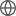Old Web
English
This paper presents a new method for calculating the cavity modes of cylindrically symmetric cavities and applies the method to stacked dielectric resonators (DRs) inside a conducting cylindrical shell. The modes of an arbitrary cavity are expressed using an eigenfunction expansion, the basis functions being the fields of an empty cavity. The wave equation for the electric displacement field, $D$ , can be manipulated to eliminate derivatives of the discontinuous dielectric constant and provide an eigenvalue equation for the resonant frequencies of the cavity. The eigenvectors specify coefficients of the empty cavity modes that sum to compose the electric and magnetic fields. A test against theory is presented for an infinitely long dielectric cylinder inside an infinitely long cylindrical cavity, and the accuracy is better than 0.4% for nearly all modes. The calculated resonant frequencies of the quasi-TE 011 (TE $_{{\mathrm {01\delta }}}$ ) mode are also compared with the experimentally measured frequencies of resonant cavities containing DRs for ten different configurations; the results display an accuracy of better than 1.0% in all but one case. A link to a MATLAB implementation of the technique is provided.